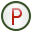# Power

The excitation of an antenna is normally specified as a complex voltage, but it may be useful to specify the total radiated or source power instead. The result is then scaled to yield the desired source power level.

Note: Power can be specified globally or per configuration.
Note:
• Feko uses peak magnitude for all complex values. Voltage and current sources must be specified with peak magnitude (as opposed to root mean square values) if no power scaling is performed.
• Power settings are specified as time-averaged values.
On the Source/Load tab, in the Settings group, click thePower icon.
No power scaling
Select this option to calculate the results using the specified source magnitudes.
Tip: A plane wave source has an infinite extent and therefore infinite power. If a model contains plane wave sources, select No power scaling.
Total source power
Select this option to scale the results such that the total source power (the sum of the power delivered by all the individual sources in a model with multiple sources) is equal to the amount specified in the Source power (Watt) field. No mismatch is taken into account.
Note: This option can be used with any source, except plane waves.
Incident power (transmission line model)

Select this option to assume that all structures are fed using transmission lines with a complex characteristic impedance Z0. The Source power field specifies the sum of the incident power from all these transmission lines. If there is a mismatch between the transmission line impedance and the structure input impedance at the excitation point, a fraction of the incident power will be reflected to the source. This is the mismatch loss.

Feko always calculates the total source power for all solutions. For large models or models with many sources, the calculation of mutual coupling (which is required for accurate source power calculations), can be time-consuming.

Select the Decouple all sources when calculating power check box to ignore the mutual coupling for Hertzian electric / magnetic dipoles or impressed line current elements when calculating the source power.

This is acceptable in the following cases:
• when sources, which in terms of the wavelength, are relatively far from each other and from other structures in the model
• when accurate power values are not required
Gain and directivity extraction are based on source power and are in general likely to be inaccurate if the Decouple all sources when calculating power option is selected.